## Linear Functions

### Learning Outcomes

• Represent a linear function with an equation, words, table and a graph.
• Determine whether a linear function is increasing, decreasing or constant.
• Calculate slope for a linear function given two points.
• Write and interpret a linear function.

Imagine placing a plant in the ground one day and finding that it has doubled its height just a few days later. Although it may seem incredible, this can happen with certain species of bamboo. These members of the grass family are the fastest growing plants in the world. One species of bamboo has been observed to grow nearly 1.5 inches every hour. In a twenty-four hour period this bamboo plant grows about 36 inches or an incredible 3 feet! A constant rate of change, such as the growth cycle of this bamboo plant, is a linear function.A bamboo forest in China. (credit: “JFXie”/Flickr)

Recall that a function is a relation that assigns to every element in the domain exactly one element in the range. A linear function is a specific type of function that can be used to model many real-world applications such as plant growth over time. In this chapter we will explore linear functions, their graphs and how to relate them to data.

## Characteristics of Linear Functions

Just as with the growth of a bamboo plant, there are many situations that involve constant change over time. For example, consider the first commercial Maglev train in the world, the Shanghai Maglev Train. It carries passengers comfortably for a 30-kilometer trip from the airport to the subway station in only 8 minutes.A view of the Shanghai Maglev Train. (credit: Rolf Wilhelm Pfennig)

Suppose that a Maglev train were to travel a long distance, and the train maintains a constant speed of 83 meters per second for a period of time once it is 250 meters from the station. How can we analyze the train’s distance from the station as a function of time? In this section, we will investigate a type of function that is useful for this purpose and use it to investigate real-world situations such as the train’s distance from the station at a given point in time.

The function describing the train’s motion is a linear function, which is defined as a function with a constant rate of change, that is, a polynomial of degree 1. There are several ways to represent a linear function including word form, function notation, tabular form and graphical form. We will describe the train’s motion as a function using each method.

### Representing a Linear Function in Word Form

Let’s begin by describing the linear function in words. For the train problem we just considered, the following word sentence may be used to describe the function relationship.

• The train’s distance from the station is a function of the time during which the train moves at a constant speed plus its original distance from the station when it began moving at a constant speed.

The speed is the rate of change. Recall that a rate of change is a measure of how quickly the dependent variable changes with respect to the independent variable. The rate of change for this example is constant, which means that it is the same for each input value. As the time (input) increases by 1 second, the corresponding distance (output) increases by 83 meters. The train began moving at this constant speed at a distance of 250 meters from the station.

### Representing a Linear Function in Function Notation

Another approach to representing linear functions is by using function notation. One example of function notation is an equation written in the form known as slope-intercept form of a line, where $x$ is the input value, $m$ is the rate of change, and $b$ is the initial value of the dependent variable. Slope-intercept form is given below:

$\begin{array}{lll}\text{Equation form}\hfill & y=mx+b\hfill \\ \text{Function notation}\hfill & f\left(x\right)=mx+b\hfill \end{array}$

In the example of the train, we might use the notation $D\left(t\right)$ in which the total distance $D$ is a function of the time $t$. The rate, $m$, is 83 meters per second. The initial value, $b$, of the dependent variable is the original distance from the station, 250 meters. We can write a generalized equation to represent the motion of the train.

$D\left(t\right)=83t+250$

### Representing a Linear Function in Tabular Form

A third method of representing a linear function is through the use of a table. The relationship between the distance from the station and the time is represented in the table below. From the table, we can see that the distance changes by 83 meters for every 1 second increase in time.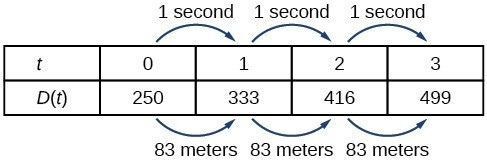Tabular representation of the function D showing selected input and output values.

### Q & A

Can the input in the previous example be any real number?

No. The input represents time, so while nonnegative rational and irrational numbers are possible, negative real numbers are not possible for this example. The input consists of nonnegative real numbers.

### Representing a Linear Function in Graphical Form

Another way to represent linear functions is visually by using a graph. We can use the function relationship from above, $D\left(t\right)=83t+250$, to draw a graph as seen below. Notice the graph is a line. When we plot a linear function, the graph is always a line.

The rate of change, which is always constant for linear functions, determines the slant or slope of the line. The point at which the input value is zero is the y-intercept of the line. We can see from the graph that the y-intercept in the train example we just saw is $\left(0,250\right)$ and represents the distance of the train from the station when it began moving at a constant speed.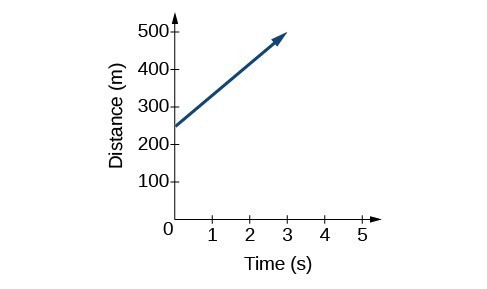The graph of $D\left(t\right)=83t+250$. Graphs of linear functions are lines because the rate of change is constant.

Notice the graph of the train example is restricted since the input value, time, must always be a nonnegative real number. However, this is not always the case for every linear function. Consider the graph of the line $f\left(x\right)=2{x}_{}+1$. Ask yourself what input values can be plugged into this function, that is, what is the domain of the function? The domain is comprised of all real numbers because any number may be doubled and then have one added to the product.

### A General Note: Linear FunctionS

A linear function is a function whose graph is a line. Linear functions can be written in slope-intercept form of a line:

$f\left(x\right)=mx+b$

where $b$ is the initial or starting value of the function (when input, $x=0$) and $m$ is the constant rate of change or slope of the function. The y-intercept is at $\left(0,b\right)$.

### Example: Using a Linear Function to MODEL the Pressure on a Diver

The pressure, $P$, in pounds per square inch (PSI) on a diver depends on depth below the water surface, $d$, in feet. This relationship may be modeled by the equation $P\left(d\right)=0.434d+14.696$. Restate this function in words.A diver exploring the depths of the ocean. (credit: Ilse Reijs and Jan-Noud Hutten)

### Determine Whether a Linear Function is Increasing, Decreasing, or Constant

The linear functions we used in the two previous examples increased over time, but not every linear function does this. A linear function may be increasing, decreasing, or constant. For an increasing function, as with the train example, the output values increase as the input values increase. The graph of an increasing function has a positive slope. A line with a positive slope slants upward from left to right as in (a). For a decreasing function, the slope is negative. The output values decrease as the input values increase. A line with a negative slope slants downward from left to right as in (b). If the function is constant, the output values are the same for all input values, so the slope is zero. A line with a slope of zero is horizontal as in (c).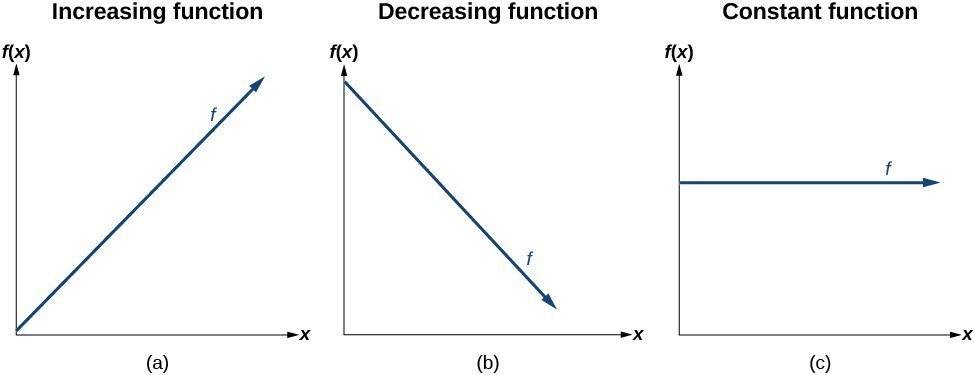### A General Note: Increasing and Decreasing Functions

The slope determines if a linear function function is an increasing, decreasing or constant.

• $f\left(x\right)=mx+b\text{ is an increasing function if }m>0$
• $f\left(x\right)=mx+b\text{ is an decreasing function if }m<0$
• $f\left(x\right)=mx+b\text{ is a constant function if }m=0$

### Example: Deciding whether a Function Is Increasing, Decreasing, or Constant

Some recent studies suggest that a teenager sends an average of 60 text messages per day. For each of the following scenarios, find the linear function that describes the relationship between the input value and the output value. Then determine whether the graph of the function is increasing, decreasing, or constant.

1. The total number of texts a teenager sends is considered a function of time in days. The input is the number of days and output is the total number of texts sent.
2. A teenager has a limit of 500 texts per month in his or her data plan. The input is the number of days and output is the total number of texts remaining for the month.
3. A teenager has an unlimited number of texts in his or her data plan for a cost of \$50 per month. The input is the number of days and output is the total cost of texting each month.

## Writing a Linear Function

### Calculate and Interpret Slope

In the examples we have seen so far, we have had the slope provided for us. However, we often need to calculate the slope given input and output values. Given two values for the input, ${x}_{1}$ and ${x}_{2}$, and two corresponding values for the output, ${y}_{1}$ and ${y}_{2}$ which can be represented by a set of points, $\left({x}_{1}\text{, }{y}_{1}\right)$ and $\left({x}_{2}\text{, }{y}_{2}\right)$, we can calculate the slope $m$, as follows:

$m=\frac{\text{change in output (rise)}}{\text{change in input (run)}}=\frac{\Delta y}{\Delta x}=\frac{{y}_{2}-{y}_{1}}{{x}_{2}-{x}_{1}}$

where $\Delta y$ is the change in output and $\Delta x$ is the change in input. In function notation, ${y}_{1}=f\left({x}_{1}\right)$ and ${y}_{2}=f\left({x}_{2}\right)$ so we could write:

$m=\frac{f\left({x}_{2}\right)-f\left({x}_{1}\right)}{{x}_{2}-{x}_{1}}$

The graph below indicates how the slope of the line between the points, $\left({x}_{1,}{y}_{1}\right)$ and $\left({x}_{2,}{y}_{2}\right)$, is calculated. Recall that the slope measures steepness. The greater the absolute value of the slope, the steeper the line is.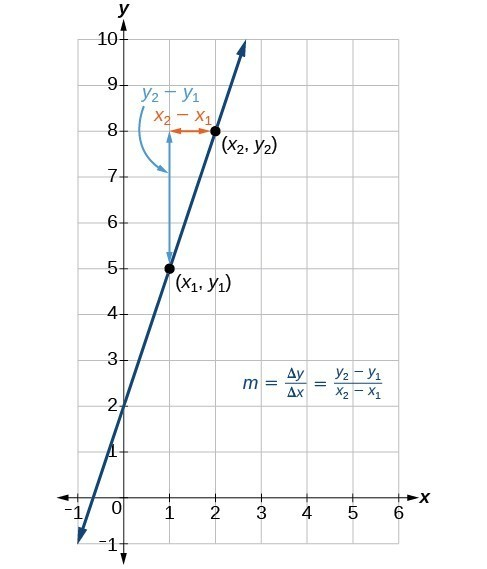The slope of a function is calculated by the change in $y$ divided by the change in $x$. It does not matter which coordinate is used as the $\left({x}_{2,\text{ }}{y}_{2}\right)$ and which is the $\left({x}_{1},\text{ }{y}_{1}\right)$, as long as each calculation is started with the elements from the same coordinate pair.

### Q & A

Are the units for slope always $\frac{\text{units for the output}}{\text{units for the input}}$ ?

Yes. Think of the units as the change of output value for each unit of change in input value. An example of slope could be miles per hour or dollars per day. Notice the units appear as a ratio of units for the output per units for the input.

### A General Note: CalculatING Slope

The slope, or rate of change, $m$, of a function can be calculated according to the following:

$m=\frac{\text{change in output (rise)}}{\text{change in input (run)}}=\frac{\Delta y}{\Delta x}=\frac{{y}_{2}-{y}_{1}}{{x}_{2}-{x}_{1}}$

where ${x}_{1}$ and ${x}_{2}$ are input values and ${y}_{1}$ and ${y}_{2}$ are output values.

### How To: Given two points from a linear function, calculate and interpret the slope.

1. Determine the units for output and input values.
2. Calculate the change of output values and change of input values.
3. Interpret the slope as the change in output values per unit of the input value.

### Example: Finding the Slope of a Linear Function

If $f\left(x\right)$ is a linear function and $\left(3,-2\right)$ and $\left(8,1\right)$ are points on the line, find the slope. Is this function increasing or decreasing?

### Try It

If $f\left(x\right)$ is a linear function, and $\left(2,3\right)$ and $\left(0,4\right)$ are points on the line, find the slope. Is this function increasing or decreasing?

### Example: Finding the Population Change from a Linear Function

The population of a city increased from 23,400 to 27,800 between 2008 and 2012. Find the change in population per year if we assume the change was constant from 2008 to 2012.

### Try It

The population of a small town increased from 1,442 to 1,868 between 2009 and 2012. Find the change in population per year if we assume the change was constant from 2009 to 2012.

### Point-Slope Form

Up until now, we have been using the slope-intercept form of a linear equation to describe linear functions. Now we will learn another way to write a linear function called point-slope form which is given below:

$y-{y}_{1}=m\left(x-{x}_{1}\right)$

where $m$ is the slope of the linear function and $({x}_{1},{y}_{1})$ is any point which satisfies the linear function.

The point-slope form is derived from the slope formula.

$\begin{array}{llll}{m}=\frac{y-{y}_{1}}{x-{x}_{1}}\hfill & \text{assuming }{ x }\ne {x}_{1}\hfill\\{ m }\left(x-{x}_{1}\right)=\frac{y-{y}_{1}}{x-{x}_{1}}\left(x-{x}_{1}\right)\hfill & \text{Multiply both sides by }\left(x-{x}_{1}\right)\hfill\\{ m }\left(x-{x}_{1}\right)=y-{y}_{1}\hfill & \text{Simplify}\hfill \\ y-{y}_{1}={ m }\left(x-{x}_{1}\right)\hfill &\text{Rearrange}\hfill \end{array}$

Keep in mind that slope-intercept form and point-slope form can be used to describe the same linear function. We can move from one form to another using basic algebra. For example, suppose we are given the equation $y - 4=-\frac{1}{2}\left(x - 6\right)$ which is in point-slope form. We can convert it to slope-intercept form as shown below.

$\begin{array}{llll}y - 4=-\frac{1}{2}\left(x - 6\right)\hfill & \hfill \\ y - 4=-\frac{1}{2}x+3\hfill & \text{Distribute the }-\frac{1}{2}.\hfill \\ \text{}y=-\frac{1}{2}x+7\hfill & \text{Add 4 to each side}.\hfill \end{array}$

Therefore, the same line can be described in slope-intercept form as $y=-\frac{1}{2}x+7$.

### A General Note: Point-Slope Form of a Linear Equation

Point-slope form of a linear equation takes the form

$y-{y}_{1}=m\left(x-{x}_{1}\right)$

where $m$ is the slope and ${x}_{1 }\text{ and } {y}_{1}$ are the $x\text{ and }y$ coordinates of a specific point through which the line passes.

Point-slope form is particularly useful if we know one point and the slope of a line. For example, suppose we are told that a line has a slope of 2 and passes through the point $\left(4,1\right)$. We know that $m=2$ and that ${x}_{1}=4$ and ${y}_{1}=1$. We can substitute these values into point-slope form.

$\begin{array}{l}y-{y}_{1}=m\left(x-{x}_{1}\right)\\ y - 1=2\left(x - 4\right)\end{array}$

If we wanted to rewrite the equation in slope-intercept form, we apply algebraic techniques.

$\begin{array}{llll} y - 1=2\left(x - 4\right)\hfill & \hfill \\ y - 1=2x - 8\hfill & \text{Distribute the }2.\hfill \\ \text{}y=2x - 7\hfill & \text{Add 1 to each side}.\hfill \end{array}$

Both equations $y - 1=2\left(x - 4\right)$ and $y=2x - 7$ describe the same line. The graph of the line can be seen below.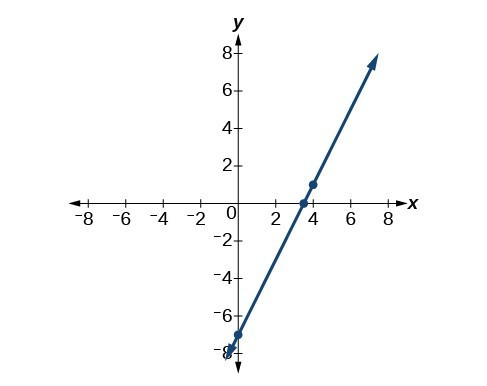### Example: Writing Linear Equations Using a Point and the Slope

Write the point-slope form of an equation of a line with a slope of 3 that passes through the point $\left(6,-1\right)$. Then rewrite the equation in slope-intercept form.

### Try It

Write the equation of a line in point-slope form with a slope of $-2$ that passes through the point $\left(-2,\text{ }2\right)$. Then rewrite the equation in the slope-intercept form.

### Writing the Equation of a Line Using Two Points

Point-slope form of an equation is also useful if we know any two points through which a line passes. Suppose, for example, we know that a line passes through the points $\left(0,\text{ }1\right)$ and $\left(3,\text{ }2\right)$. We can use the coordinates of the two points to find the slope.

$\begin{array}{l}{m}=\frac{{y}_{2}-{y}_{1}}{{x}_{2}-{x}_{1}}\\ \text{}{m}=\frac{2 - 1}{3 - 0}\hfill \\ \text{}{m}=\frac{1}{3}\hfill \end{array}$

Now we can use the slope we found and the coordinates of one of the points to find the equation for the line. Let’s use (0, 1) for our point.

$\begin{array}{l}y-{y}_{1}=m\left(x-{x}_{1}\right)\\ y - 1=\frac{1}{3}\left(x - 0\right)\end{array}$

As before, we can use algebra to rewrite the equation in slope-intercept form.

$\begin{array}{lll}y - 1=\frac{1}{3}\left(x - 0\right)\hfill & \hfill \\ y - 1=\frac{1}{3}x\hfill & \text{Distribute the }\frac{1}{3}.\hfill \\ \text{}y=\frac{1}{3}x+1\hfill & \text{Add 1 to each side}.\hfill \end{array}$

Both equations describe the line graphed below.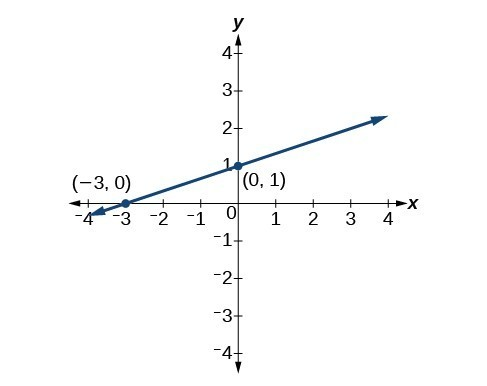### Example: Writing Linear Equations Using Two Points

Write the point-slope form of an equation of a line that passes through the points $\left(5,\text{ }1\right)$ and $\left(8,\text{ }7\right)$. Then rewrite the equation in slope-intercept form.

### Try It

Write the point-slope form of an equation of a line that passes through the points $\left(-1,3\right)$ and $\left(0,0\right)$. Then rewrite the equation in slope-intercept form.

## Key Equations

 slope-intercept form of a line $y=mx+b$ slope $m=\frac{\text{change in output (rise)}}{\text{change in input (run)}}=\frac{\Delta y}{\Delta x}=\frac{{y}_{2}-{y}_{1}}{{x}_{2}-{x}_{1}}$ point-slope form of a line $y-{y}_{1}=m\left(x-{x}_{1}\right)$

## Key Concepts

• The ordered pairs given by a linear function represent points on a line.
• Linear functions can be represented in words, function notation, tabular form and graphical form.
• The rate of change of a linear function is also known as the slope.
• An equation in slope-intercept form of a line includes the slope and the initial value of the function.
• The initial value, or y-intercept, is the output value when the input of a linear function is zero. It is the y-value of the point where the line crosses the y-axis.
• An increasing linear function results in a graph that slants upward from left to right and has a positive slope.
• A decreasing linear function results in a graph that slants downward from left to right and has a negative slope.
• A constant linear function results in a graph that is a horizontal line.
• Analyzing the slope within the context of a problem indicates whether a linear function is increasing, decreasing, or constant.
• The slope of a linear function can be calculated by dividing the difference between y-values by the difference in corresponding x-values of any two points on the line.
• The slope and initial value can be determined given a graph or any two points on the line.
• One form of a linear function is slope-intercept form.
• Point-slope form is useful for finding the equation of a linear function when given the slope of a line and one point.
• Point-slope form is also convenient for finding the equation of a linear function when given two points through which a line passes.
• The equation for a linear function can be written in slope-intercept form if the slope m and initial value are known.
• A linear function can be used to solve real-world problems.
• A linear function can be written from tabular form.

## Glossary

decreasing linear function
a function with a negative slope: If $m<0, \text{then }f\left(x\right)=mx+b$ is decreasing.
increasing linear function
a function with a positive slope: If $m>0, \text{then }f\left(x\right)=mx+b$ is increasing.
linear function
a function with a constant rate of change that is a polynomial of degree 1 whosegraph is a straight line
point-slope form
the equation of a linear function of the form $y-{y}_{1}=m\left(x-{x}_{1}\right)$
slope
the ratio of the change in output values to the change in input values; a measure of the steepness of a line
slope-intercept form
the equation of a linear function of the form $f\left(x\right)=mx+b$
y-intercept
the value of a function when the input value is zero; also known as initial value